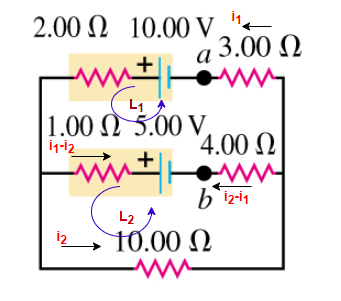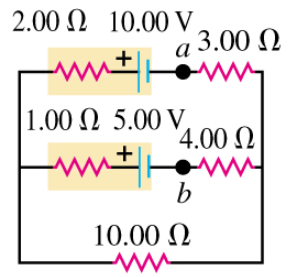# Problem: A. In the circuit shown in the figure, find the magnitude of current in the upper branch.B. Find the magnitude of current in the middle branch.C. Find the magnitude of current in the lower branch.D. What is the potential difference Vab of point a relative to point b?

###### FREE Expert Solution

Kirchhoff's loop rule:

$\overline{){\mathbf{\Sigma }}{\mathbf{V}}{\mathbf{=}}{\mathbf{0}}}$A. Considering the upper loop:

Applying Kirchhoff's loop rule in L1

$\begin{array}{rcl}\begin{array}{rc}{\mathbf{i}}_{\mathbf{1}}\mathbf{3}\mathbf{.}\mathbf{00}\mathbf{\Omega }& \mathbf{-}\mathbf{10}\mathbf{.}\mathbf{00}\mathbf{V}\mathbf{+}{\mathbf{i}}_{\mathbf{1}}\mathbf{2}\mathbf{.}\mathbf{00}\mathbf{\Omega }\mathbf{+}\mathbf{\left(}{\mathbf{i}}_{\mathbf{1}}\mathbf{-}{\mathbf{i}}_{\mathbf{2}}\mathbf{\right)}\mathbf{1}\mathbf{.}\mathbf{00}\mathbf{\Omega }\mathbf{+}\mathbf{5}\mathbf{.}\mathbf{00}\mathbf{V}\mathbf{-}\mathbf{\left(}{\mathbf{i}}_{\mathbf{2}}\mathbf{-}{\mathbf{i}}_{\mathbf{1}}\mathbf{\right)}\mathbf{4}\mathbf{.}\mathbf{00}\mathbf{\Omega }\end{array}& \mathbf{=}& \mathbf{0}\\ {\mathbf{i}}_{\mathbf{1}}\mathbf{5}\mathbf{.}\mathbf{00}\mathbf{\Omega }\mathbf{+}{\mathbf{i}}_{\mathbf{1}}\mathbf{1}\mathbf{.}\mathbf{00}\mathbf{\Omega }\mathbf{-}{\mathbf{i}}_{\mathbf{2}}\mathbf{1}\mathbf{.}\mathbf{00}\mathbf{\Omega }\mathbf{-}{\mathbf{i}}_{\mathbf{2}}\mathbf{4}\mathbf{.}\mathbf{00}\mathbf{\Omega }\mathbf{+}{\mathbf{i}}_{\mathbf{1}}\mathbf{4}\mathbf{.}\mathbf{00}\mathbf{\Omega }\mathbf{-}\mathbf{5}\mathbf{.}\mathbf{00}\mathbf{V}& \mathbf{=}& \mathbf{0}\\ {\mathbf{i}}_{\mathbf{1}}\mathbf{10}\mathbf{.}\mathbf{00}\mathbf{\Omega }\mathbf{-}{\mathbf{i}}_{\mathbf{2}}\mathbf{5}\mathbf{.}\mathbf{00}\mathbf{\Omega }\mathbf{-}\mathbf{5}\mathbf{.}\mathbf{00}\mathbf{V}& \mathbf{=}& \mathbf{0}\\ {\mathbf{i}}_{\mathbf{1}}\mathbf{10}\mathbf{.}\mathbf{00}\mathbf{\Omega }\mathbf{-}{\mathbf{i}}_{\mathbf{2}}\mathbf{5}\mathbf{.}\mathbf{00}\mathbf{\Omega }& \mathbf{=}& \mathbf{5}\mathbf{.}\mathbf{00}\mathbf{V}\end{array}$

88% (61 ratings)###### Problem DetailsA. In the circuit shown in the figure, find the magnitude of current in the upper branch.

B. Find the magnitude of current in the middle branch.

C. Find the magnitude of current in the lower branch.

D. What is the potential difference Vab of point a relative to point b?